# Planimetrics - math word problems

Study plane measurements, including angles, distances, and areas. In other words - measurement and calculation of shapes in the plane. Perimeter and area of plane shapes.

#### Number of problems found: 1947

• Is right triangleDecide if the triangle XYZ is rectangular: x = 4 m, y = 6 m, z = 4 m
• Vertices of RTShow that the points P1 (5,0), P2 (2,1) & P3 (4,7) are the vertices of a right triangle.
• GardenHow many steps of 76 cm circumvent square garden with area 1.8 ha?
• Trapezium zoomHow many times increase area of trapezoid if all sides and altitude increase 5 times?Find the radius of the circle using the Pythagorean theorem where a=9, b=r, c= 6+r
• How manyHow many different rectangles with integer page lengths have an area S = 60 cm²?
• CalculateCalculate the area of the ABE triangle AB = 38mm and height E = 42mm ps: please try a quick calculation
• Triangle ABCTriangle ABC has side lengths m-1, m-2, m-3. What has to be m to be triangle a) rectangular b) acute-angled?
• Type of triangle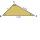How do I find the triangle type if the angle ratio is 2:3:7 ?
• Cross five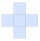The figure on the picture is composed of the same squares and has a content of 45cm². What's its perimeter?
• Hexagon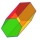Calculate the surface of a regular hexagonal prism whose base edge a = 12cm and side edge b = 3 dm.
• Fence of the garden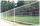The garden has dimensions of 5m and 400cm. How many meters meshes are needed for fencing the plot?
• Triangle perimeterCalculate the triangle perimeter whose sides are in ratio 3: 5: 7 and the longest side is 17.5 cm long.
• Square and circlesSquare with sides 83 cm is circumscribed and inscribed with circles. Determine the radiuses of both circles.
• Square side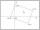Calculate length of side square ABCD with vertex A[0, 0] if diagonal BD lies on line p: -4x -5 =0.
• Integer sidesA right triangle with an integer length of two sides has one leg √11 long. How much is its longest side?
• Two triangles SSA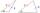Two triangles can be formed with the given information. Use the Law of Sines to solve the triangles. A = 59°, a = 13, b = 14
• OctagonWe have a square with side 84 cm. We cut the corners to make his octagon. What will be the side of the octagon?
• Coordinates of square verticesThe ABCD square has the center S [−3, −2] and the vertex A [1, −3]. Find the coordinates of the other vertices of the square.
• Chord - TS v2The radius of circle k measures 87 cm. Chord GH = 22 cm. What is TS?

Do you have an interesting mathematical word problem that you can't solve it? Submit a math problem, and we can try to solve it.

We will send a solution to your e-mail address. Solved examples are also published here. Please enter the e-mail correctly and check whether you don't have a full mailbox.

Please do not submit problems from current active competitions such as Mathematical Olympiad, correspondence seminars etc...